# SAT Math Multiple Choice Question 54: Answer and Explanation

### Test Information

Question: 54

4.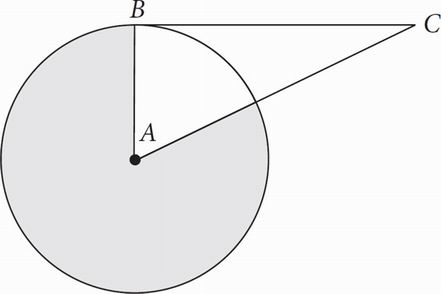Note:Figure not drawn to scale.

The circle above with center A has an area of 21. BC is tangent to the circle with center A at point B. If AC = 2AB, then what is the area of the shaded region?

• A. 3.5
• B. 15.75
• C. 17.5
• D. 21

C The trick is to recognize that DABC is a 30°-60°-90° right triangle. ∠ABC must equal 90° since a tangent line must be perpendicular to the radius of a circle drawn to the point of tangency. Only a 30°-60°-90° has a hypotenuse (AC) equal to double the length of one of the sides (AB). (You can also use the Pythagorean theorem to show this.) This means that ∠BAC = 60°, so the shaded region has a central angle measure of 360° - 60° = 300°. To get the area, use the proportion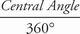=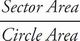, or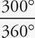=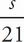. Reduce, cross-multiply, and solve to get s = 17.5. This matches (C).